﻿ 采用直翼推进器的舰船动力装置仿真
 舰船科学技术2019, Vol. 41Issue (2): 93-98PDF

Simulation study on the power installation of warship with cycloidal propellers
HE Qing, LIU Jin-lin, ZENG Fan-ming
College of Power Engineering, Naval University of Engineering, Wuhan 430033, China
Abstract: The size and direction of thrust can be changed quickly when cycloidal propeller is running, and the propeller has excellent maneuverability and mobility. So cycloidal propeller is used widely in special ships, such as tugs, minesweepers and ocean engineering platform.This paper takes the ship with cycloidal propellers as research object. By studying the energy conversion between ship, diesel engine and propellers, and using MMG model, the Matlab/Simulink model of system is established. The matching of system can be studied on further step via running the Simulink model, and then providing references for designing and manufacturing special ship with cycloidal propellers.
Key words: the matching of hull-diesel engine-propeller     cycloidal propeller     MMG     Matlab/Simulink
0 引　言

1 直翼推进器动力装置简介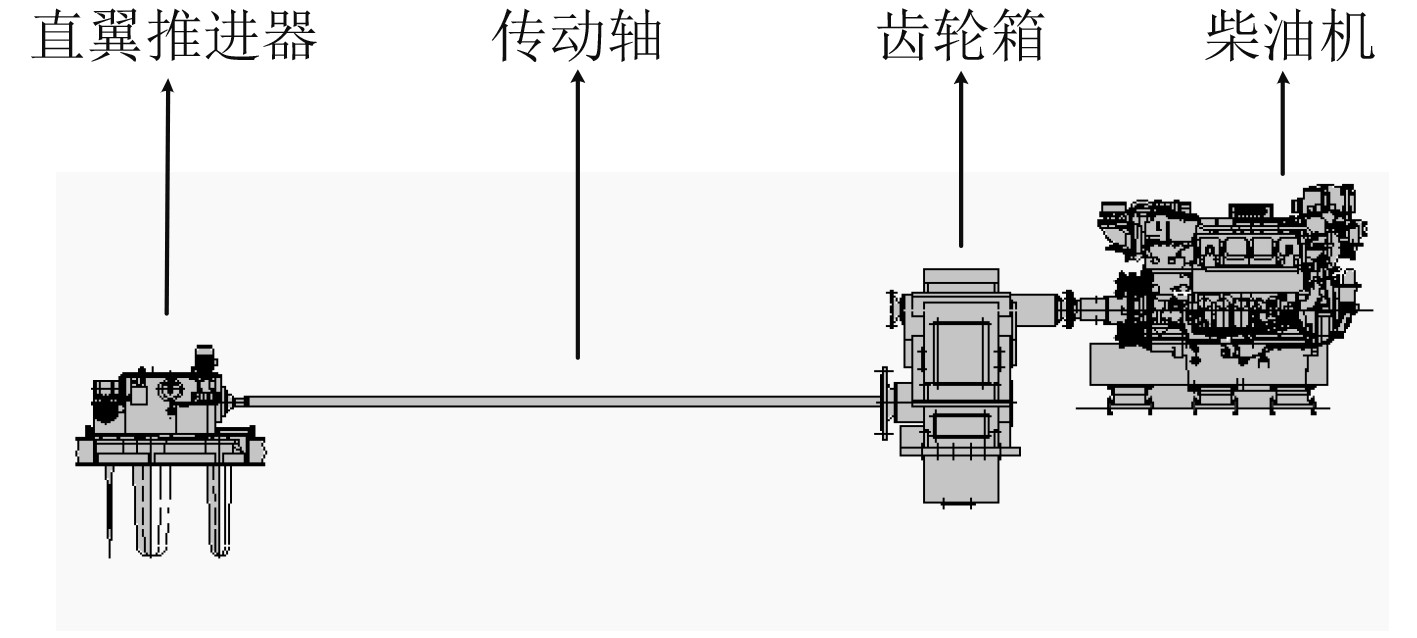图 1 某直翼推进器动力装置简图 Fig. 1 The propulsion system with cycloidal propeller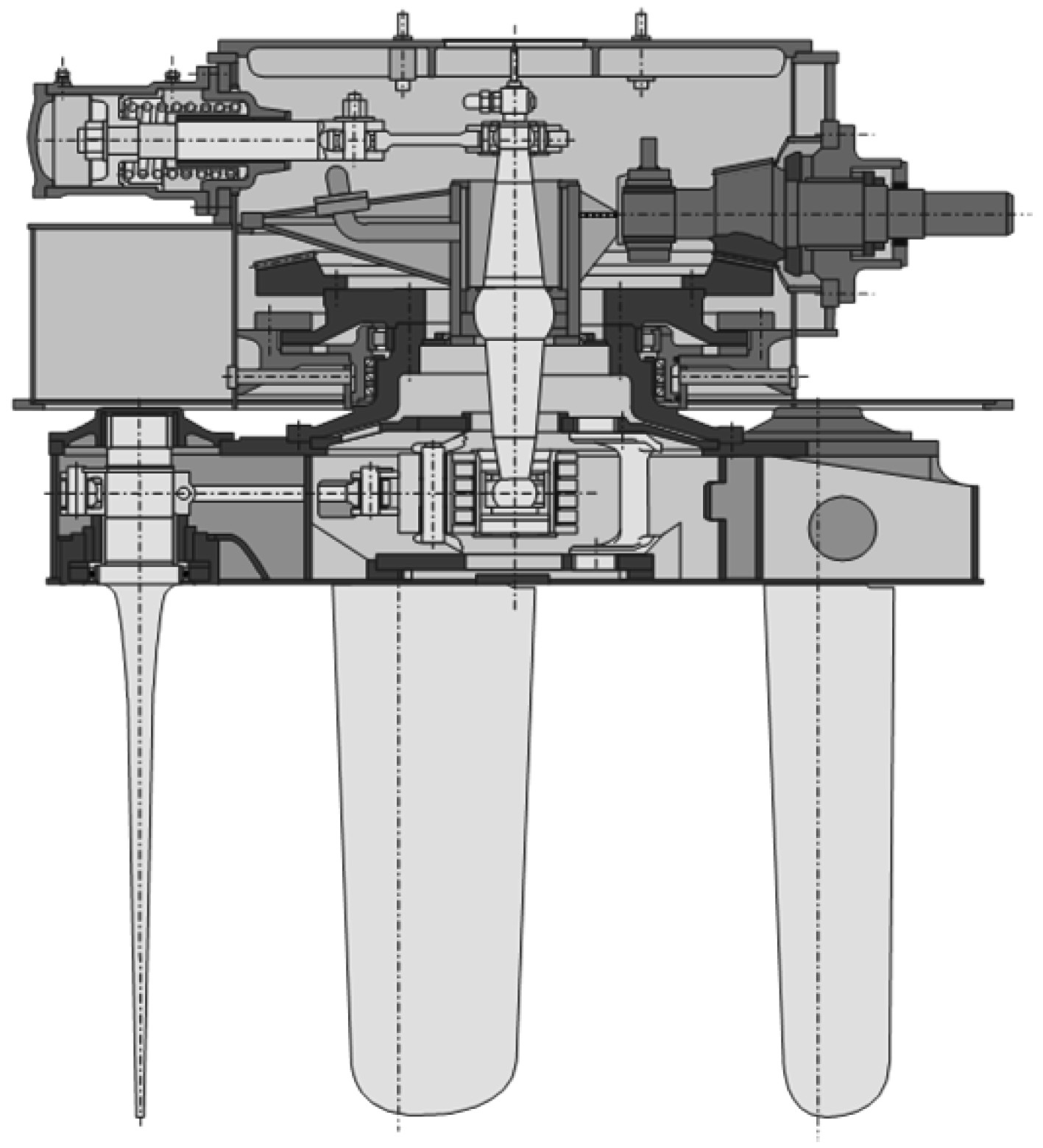图 2 直翼推进器结构图 Fig. 2 The structure of cycloidal propeller
2 系统数学模型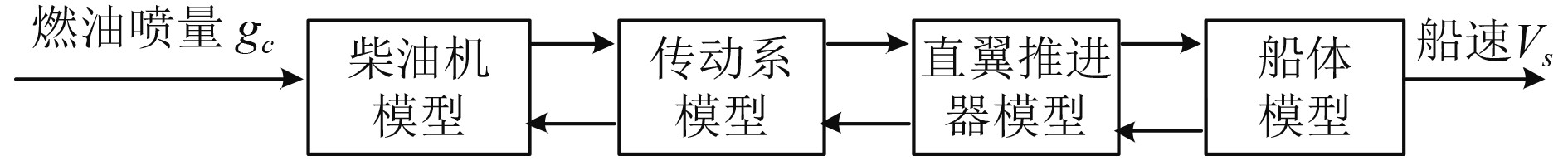图 3 船机桨匹配模型结构简图 Fig. 3 The model of ship-diesel-propeller matching

 $\left\{ \begin{split} & {{M}_{D}}i-{{M}_{f}}-{{M}_{P}}=\left( J+{{J}_{S}} \right)\displaystyle\frac{{\rm{d}}{{\omega }_{P}}}{{\rm{d}}t}{\text{，}} \\ & {{n}_{D}}={{n}_{P}}i{\text{。}} \\ \end{split} \right.$

 $\left\{ \begin{split} & {{Z}_{P}}T\left( 1-{{t}_{P}} \right)-R=\left( m+{{m}_{s}} \right)\displaystyle\frac{d{{V}_{S}}}{{\rm{d}}\rm{t}}{\text{，}} \\ & {{V}_{A}}=\left( 1-\omega \right){{V}_{S}} {\text{。}} \\ \end{split} \right.$

2.1 柴油机模型

2.2 直翼推进器数学模型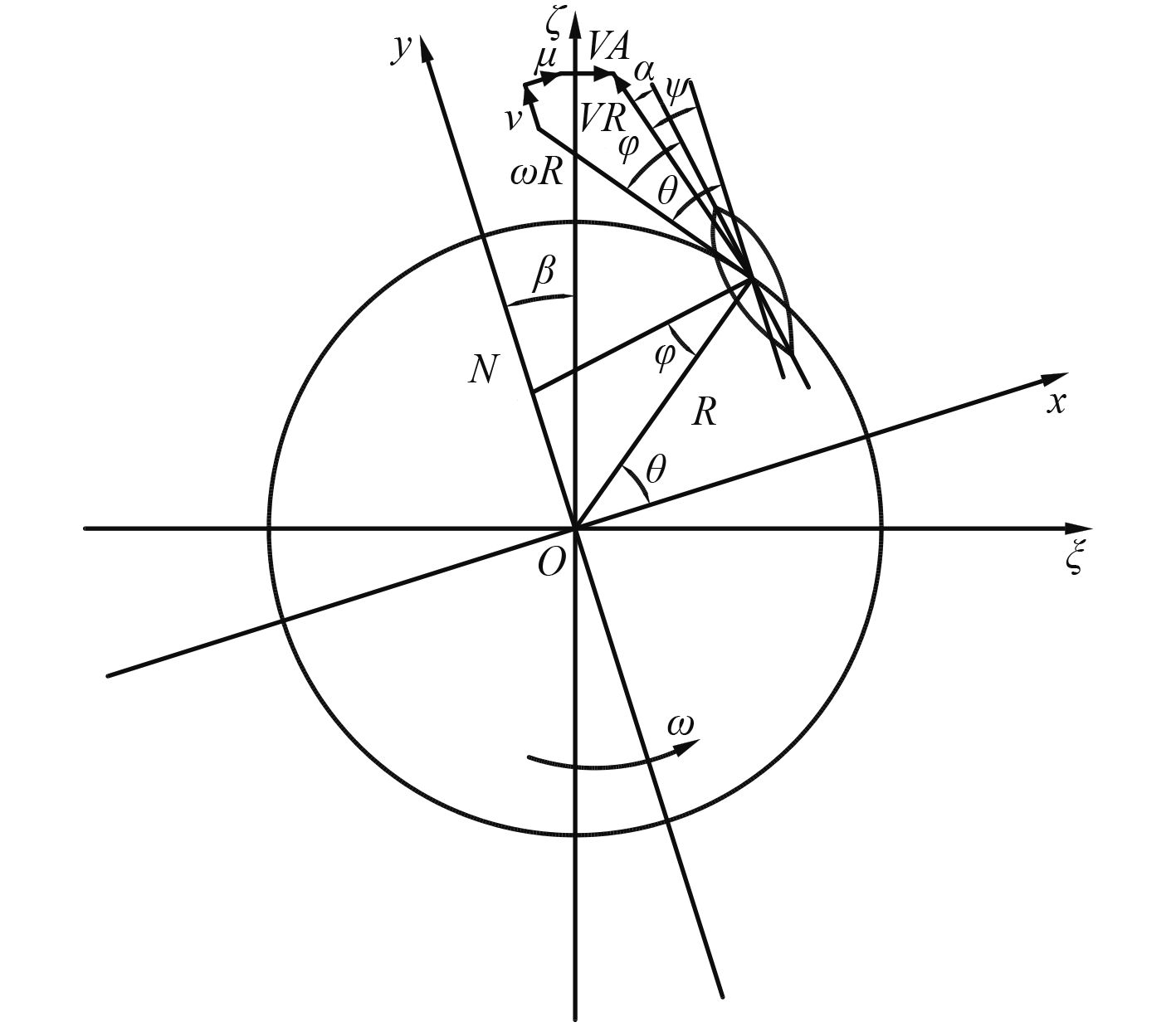图 4 水动力分析图 Fig. 4 The analysis of force
 $\left\{ \begin{split} & {{t}_{X}}\left( \theta \right)=L\cos \left( \theta -\varphi +\alpha \right)+D\sin \left( \theta -\varphi +\alpha \right) {\text{，}} \\ & {{t}_{Y}}\left( \theta \right)=L\sin \left( \theta -\varphi +\alpha \right)-D\cos \left( \theta -\varphi +\alpha \right) {\text{，}} \\ & m\left( \theta \right)=\left[ L\sin \left( \varphi -\alpha \right)+D\cos \left( \varphi -\alpha \right) \right]R{\text{。}} \end{split} \right.$

 \begin{aligned} & {T_X} = \frac{Z}{{4{\text{π}} }}\rho cb\int_{ - \text{π} }^\text{π} {{V_R}} \left[ \begin{aligned} & {C_L}\left( {\omega R\cos (\theta ) + (v - {V_A}\sin (\beta ))} \right) + \\ & {C_D}\left( {\omega R\sin (\theta ) - (u + {V_A}\cos (\beta ))} \right) \end{aligned} \right]{\rm{d}}\theta {\text{，}}\\ & {T_Y} = \frac{Z}{{4\text{π} }}\rho cb\int_{ - \text{π} }^\text{π} {{V_R}} \left[ \begin{aligned} & {C_L}\left( {\omega R\sin (\theta ) - (u + {V_A}\cos (\beta ))} \right) + \\ & {C_D}\left( {\omega R\cos (\theta ) + (v - {V_A}\sin (\beta ))} \right) \end{aligned} \right]{\rm{d}}\theta{\text{，}} \\ & \begin{aligned} & M = \frac{Z}{{4\text{π} }}\rho cb\int_{ - \text{π} }^\text{π} {{V_R}}\;\times\\ & \left\{ \begin{aligned} & {C_L}\left[ {(u + {V_A}\cos (\beta ))\cos (\theta ) + (v - {V_A}\sin (\beta ))\sin (\theta )} \right] + \\ & {C_D}\left[ {\omega R - (u + {V_A}\cos (\beta ))\sin (\theta ) + (v - {V_A}\sin (\beta ))\cos (\theta \!)} \right] \end{aligned}\! \right\}{\rm{d}}\theta {\text{，}} \end{aligned} \end{aligned}

 ${{T}_{P}}=\sqrt{T_{X}^{2}+T_{Y}^{2}}{\text{，}}$

${T_X}$ ${T_Y}$ $M$ 进行无因次化，推力系数KXKY和转矩系数KM计算如下：

 $\left\{ \begin{split} {{K}_{X}}=\displaystyle\frac{{{T}_{X}}}{\rho n_{P}^{2}D_{P}^{3}b}{\text{，}} \\ {{K}_{Y}}=\displaystyle\frac{{{T}_{Y}}}{\rho n_{P}^{2}D_{P}^{3}b}{\text{，}} \\ {{K}_{M}}=\displaystyle\frac{M}{\rho n_{P}^{2}D_{P}^{4}b}{\text{。}} \\ \end{split} \right.$

 $\left\{ \begin{matrix} {{K}_{T}}={{K}_{X}}\cos \beta -{{K}_{Y}}\sin \beta {\text{，}} \\ {{K}_{S}}={{K}_{X}}\sin \beta +{{K}_{Y}}\cos \beta {\text{。}} \\ \end{matrix} \right.$

 $\eta =\frac{{{V}_{A}}T}{2{\text{π}} nM}=\frac{J}{2{\text{π}} }\cdot \frac{{{K}_{T}}}{{{K}_{S}}}{\text{。}}$

2.3 船体数学模型 2.3.1 伴流系数和推力减额系数估算

1）伴流系数估算。

 ${{\omega }_{p0}}=0.7{{C}_{P}}-0.3{\text{。}}$

2）推力减额系数估算

①汉克歇尔估算式

 ${{t}_{p0}}=0.5{{C}_{P}}-0.18{\text{；}}$

②哥铁堡公式

 ${{t}_{p0}}=1.67-2.3\frac{{{C}_{b}}}{{{C}_{WP}}}+1.5{{C}_{b}}{\text{。}}$

2.3.2 船体运动模型

 $\left\{ \begin{split} & m\left( \dot{u}-vr \right)={{X}_{I}}+{{X}_{H}}+{{X}_{P}}{\text{，}} \\ & m\left( \dot{v}+ur \right)={{Y}_{I}}+{{Y}_{H}}+{{Y}_{P}}{\text{，}} \\ & {{I}_{Z}}\dot{r}={{M}_{I}}+{{M}_{H}}+{{M}_{P}} {\text{。}} \\ \end{split} \right.$

1）船体惯性类水动力和力矩。由周昭明对元良诚三图谱进行多元回归分析，其估算公式如下

 \begin{split} &{\displaystyle\frac{{{m_x}}}{m} = \frac{1}{{100}}\left[ \begin{aligned} & 0.398 + 11.97{C_b}\left(1 + 3.73\frac{d}{B}\right) - 2.89{C_b}\frac{L}{B} \times \\ &\left(1 + 1.13\frac{d}{B}\right) + 0.175{C_b}{\left(\displaystyle\frac{L}{B}\right)^2}\left(1 + 0.541\frac{d}{B}\right)- \\ & 1.107\frac{L}{B}\frac{d}{B} \end{aligned} \right]}{\text{，}}\\ &{\displaystyle\frac{{{m_y}}}{m} = 0.882 - 0.54{C_b}\left(1 - 1.6\frac{d}{B}\right) - 0.156\frac{L}{B}(1 - 0.673{C_b})}+\\ &\quad{ 0.826\frac{d}{B}\frac{L}{B}\left(1 - 0.678\frac{d}{B}\right) - 0.638{C_b}\displaystyle\frac{d}{B}\frac{L}{B}\left(1 - 0.669\frac{d}{B}\right)}\times\\ &{\sqrt {\displaystyle\frac{{{J_Z}}}{m}} = \frac{L}{{100}}\left[\begin{aligned} & 33 - 76.85{C_b}\left(1 - 0.784{C_b}\right)\\ & + 3.43\displaystyle\frac{L}{B}\left(1 - 0.63{C_b}\right) \end{aligned}\right]} {\text{。}}\end{split}

2）船体粘性类水动力和力矩。目前对粘性水动力计算模型是按漂角β的大小选取的，本文选择贵岛模型进行计算：

 \left\{ \begin{aligned} & {{X}_{H}}=X\left( u \right)+{{X}_{vv}}{{v}^{2}}+{{X}_{vr}}vr+{{X}_{rr}}rr {\text{，}} \\ & {{Y}_{H}}={{Y}_{v}}v+{{Y}_{r}}r+{{Y}_{vv}}\left| v \right|v+{{Y}_{rr}}\left| r \right|r+{{Y}_{vvr}}{{v}^{2}}+{{Y}_{vrr}}v{{r}^{2}}{\text{，}} \\ & {{M}_{H}}={{M}_{v}}+{{M}_{r}}r+{{M}_{vv}}\left| v \right|v+{{M}_{rr}}\left| r \right|r+{{M}_{vvr}}{{v}^{2}}r+{{M}_{vrr}}v{{r}^{2}} {\text{。}}\end{aligned} \right.

3）直翼推进器推力、横向力和力矩。直翼推进器具有优良的操纵性，其纵向推力是主动力，横向推力是起到舵的作用。图5为推进器控制点N在任意位置与推力关系的示意图。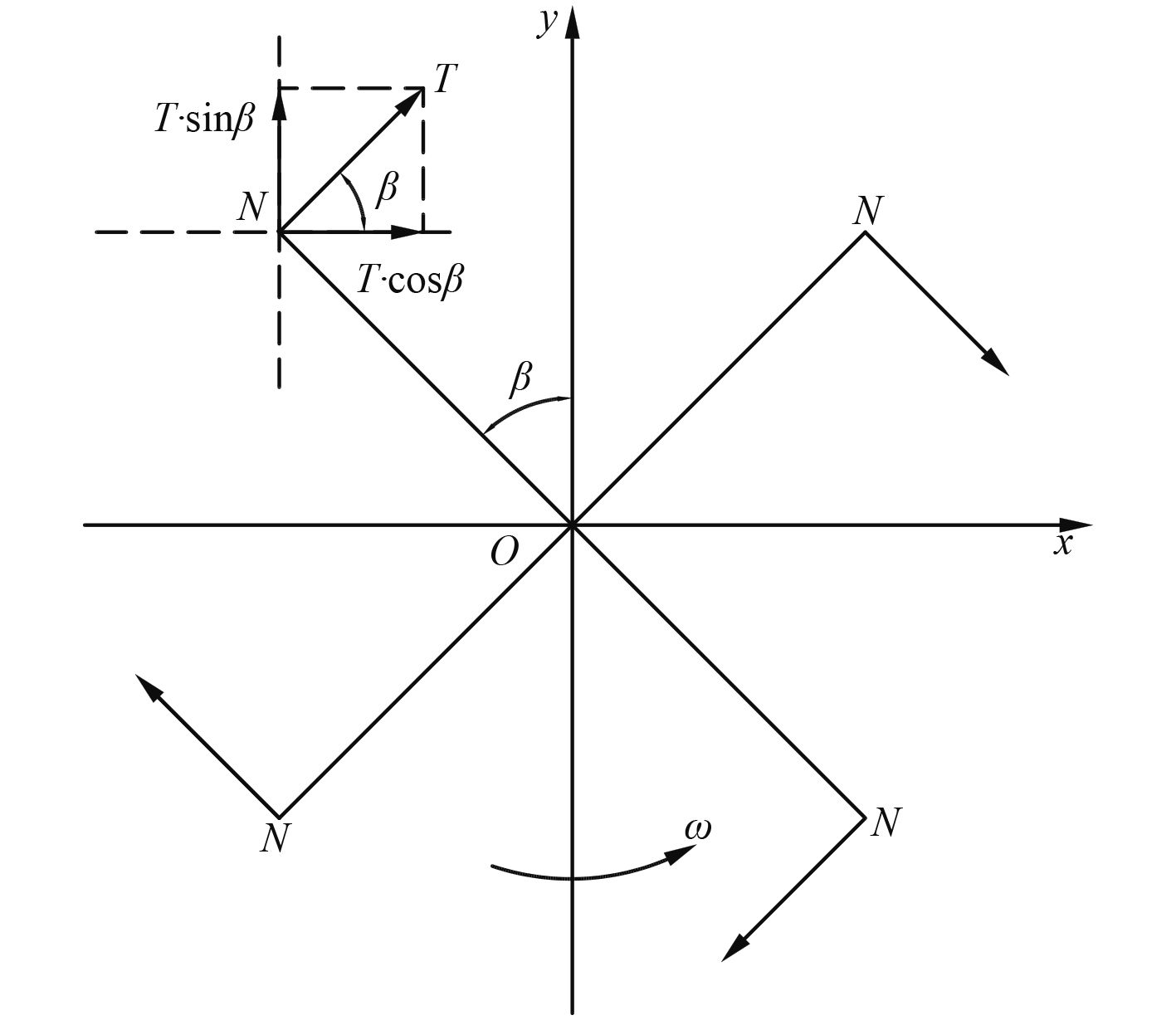图 5 N点位置与推力方向示意图 Fig. 5 Relation between position N and thrust direction

 $\left\{ \begin{matrix} {{T}_{x}}=T\cos \beta {\text{，}} \\ {{T}_{y}}=T\sin \beta {\text{，}} \\ \end{matrix} \right.$

 ${{T}_{P}}=\left( 1-{{t}_{P}} \right)\rho n_{P}^{2}D_{P}^{3}{{L}_{P}}{{K}_{t}}\left( {{J}_{P}} \right){\text{。}}$

 ${{T}_{S}}=\left( 1-{{t}_{P}} \right)\rho n_{S}^{2}D_{P}^{3}{{L}_{P}}{{K}_{t}}\left( {{J}_{P}} \right){\text{。}}$

 \left\{ \begin{aligned} & {{X}_{P}}={{T}_{P}}\cos \left( {{\delta }_{P}} \right)+{{T}_{S}}\cos \left( {{\delta }_{S}} \right){\text{，}} \\ & {{Y}_{P}}={{T}_{P}}\sin \left( {{\delta }_{P}} \right)+{{T}_{S}}\sin \left( {{\delta }_{S}} \right){\text{，}} \\ & {{M}_{P}}=\left( {{T}_{P}}\cos \left( {{\delta }_{P}} \right)-{{T}_{S}}\cos \left( {{\delta }_{S}} \right) \right)\frac{{{L}_{PS}}}{2}-{{Y}_{P}}\frac{{{L}_{OP}}}{2}{\text{，}} \\ & {{K}_{P}}={{Y}_{P}}{{Z}_{P}} {\text{。}} \end{aligned} \right.

 \left\{ \begin{aligned} & \psi \left( t \right)=\int_{0}^{t}{r{\rm{d}}t}{\text{，}} \\ & x=\int_{0}^{t}{{{V}_{x}}{\rm{d}}t}=\int_{0}^{t}{\left( u\cos \psi -v\sin \psi \right){\rm{d}}t}{\text{，}} \\ & y=\int_{0}^{t}{{{V}_{y}}{\rm{d}}t}=\int_{0}^{t}{\left( u\sin \psi +v\cos \psi \right){\rm{d}}t} {\text{。}} \end{aligned} \right.
3 船体-主机-推进器仿真模型 3.1 柴油机模型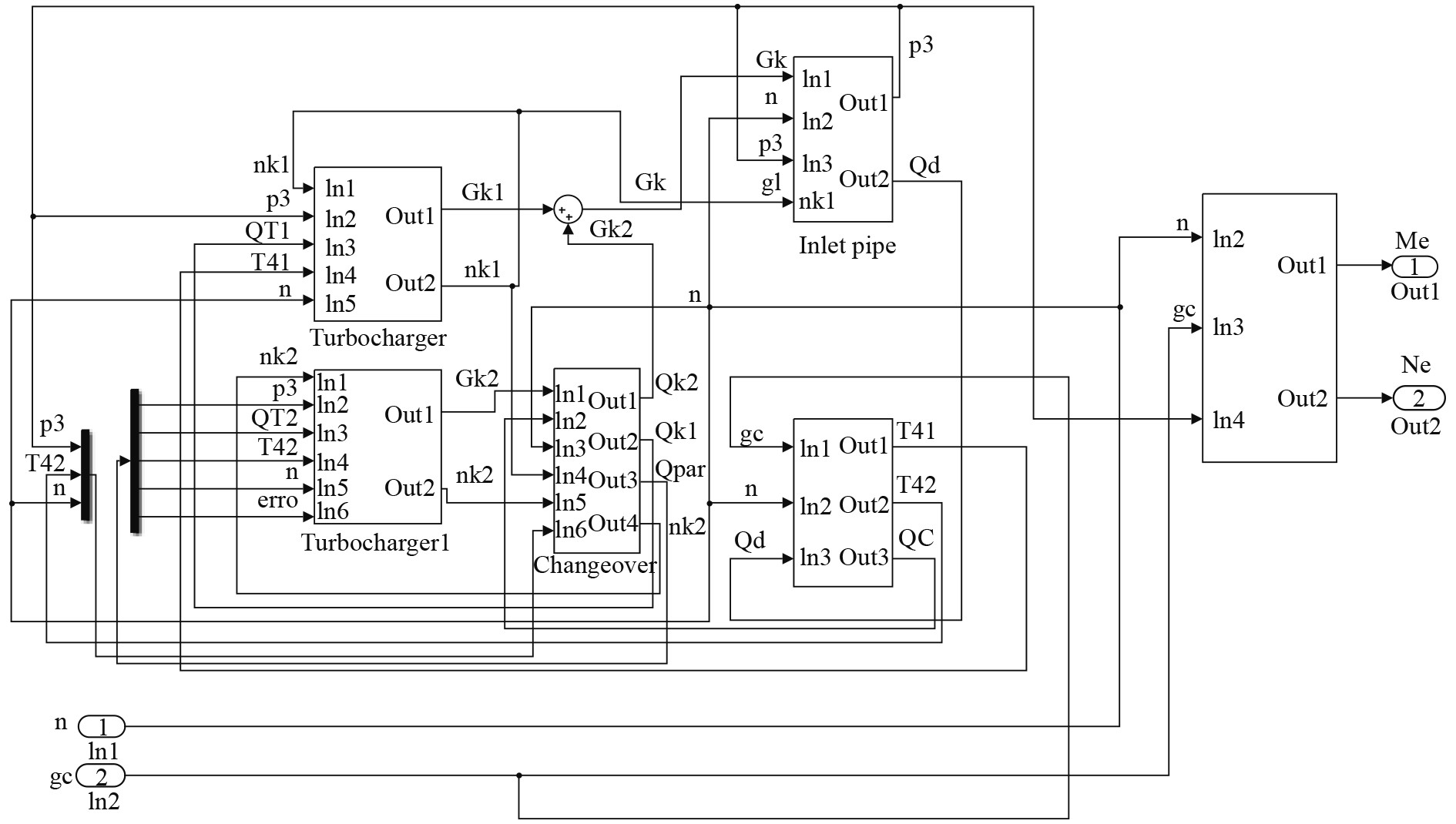图 6 柴油机Simulink模型图 Fig. 6 The Simulink model of diesel
3.2 直翼推进器模型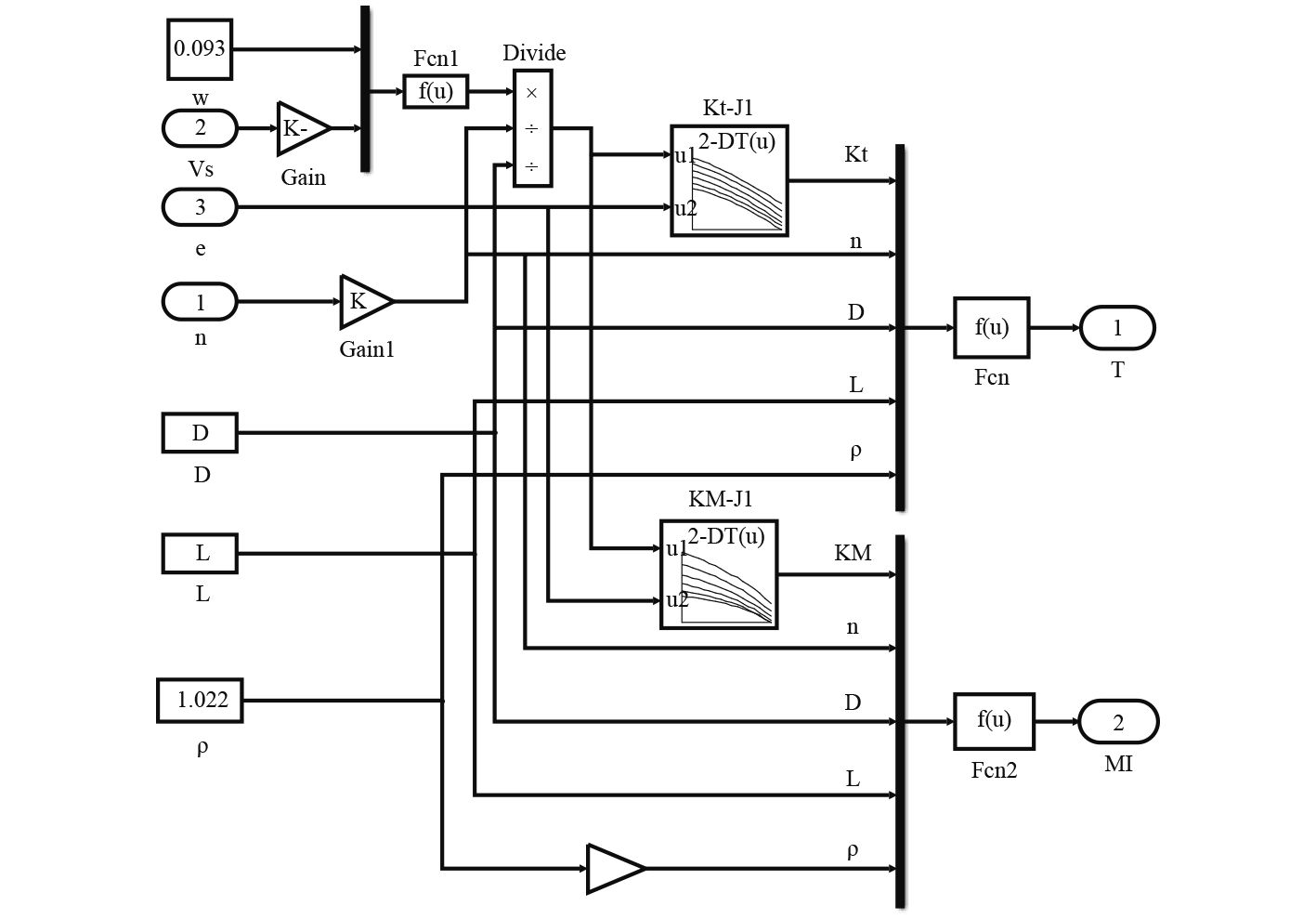图 7 直翼推进器Simulink模型图 Fig. 7 The Simulink model of cycloidal propeller
3.3 船体运动模型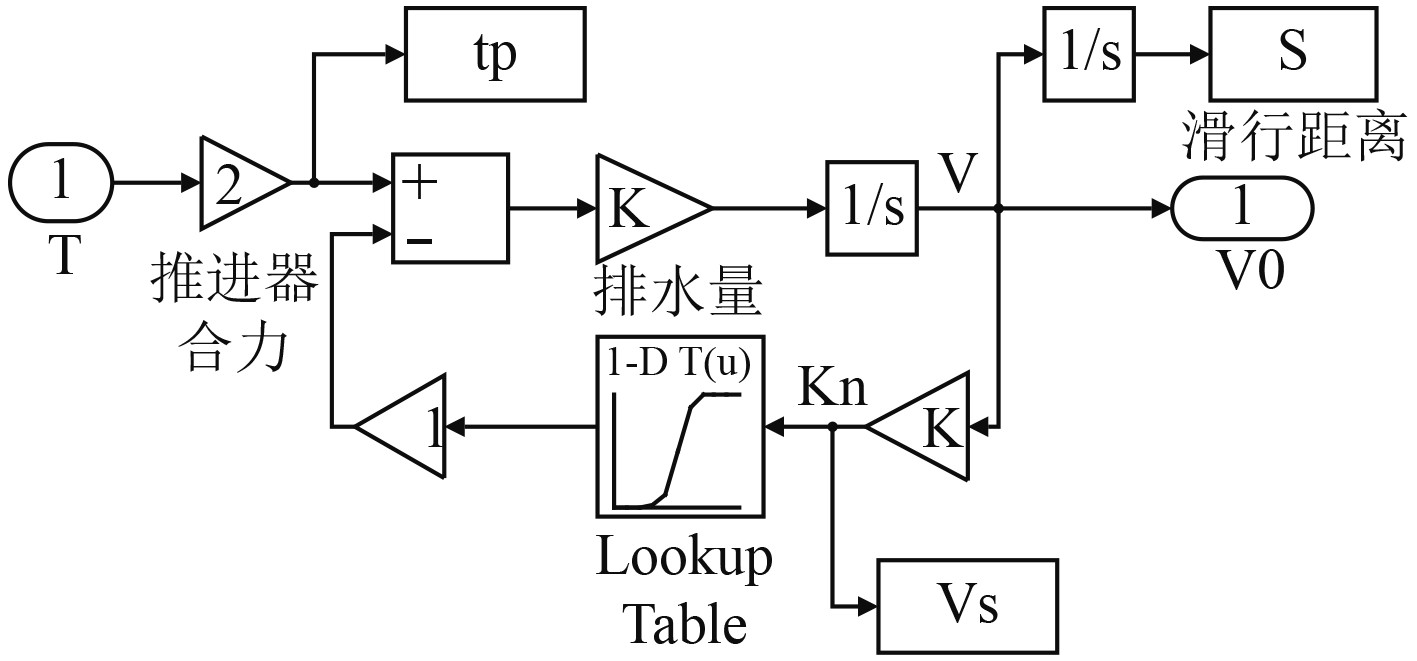图 8 基于Simulink船舶直航运动仿真模型 Fig. 8 The Simulink model of ship motion
3.4 齿轮箱及轴系模型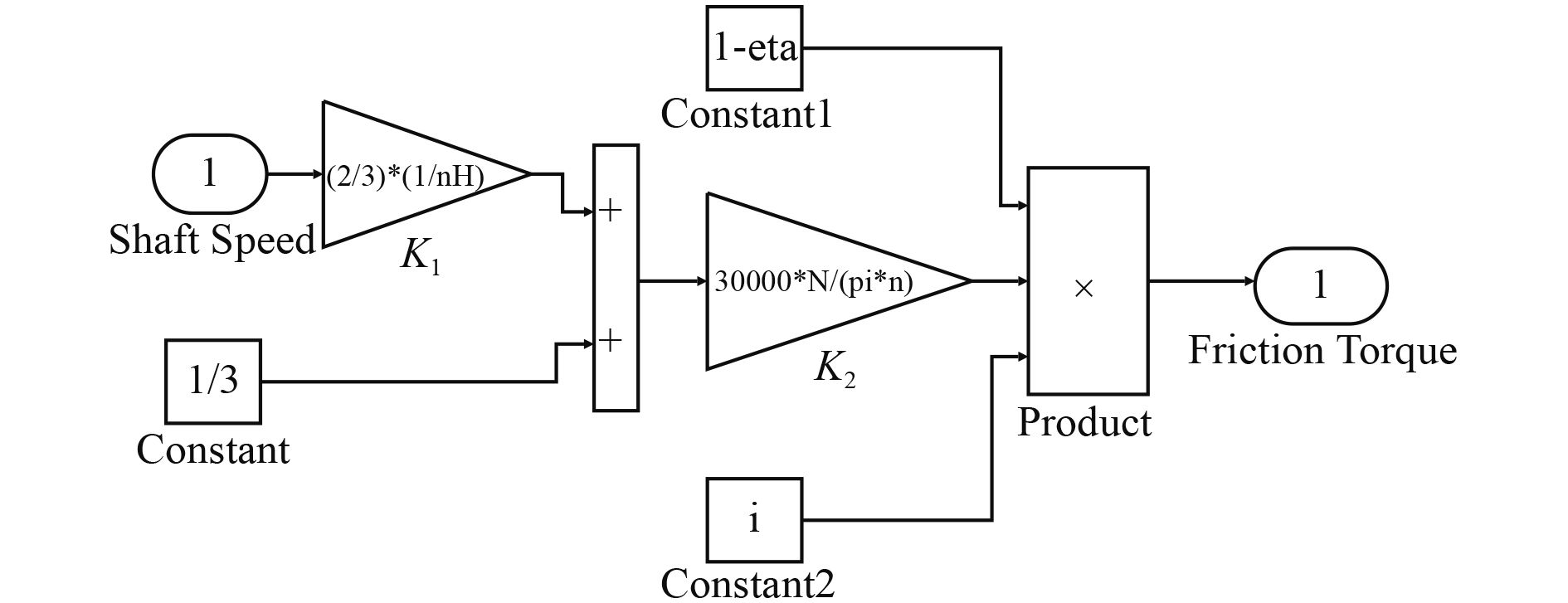图 9 轴系摩擦损失仿真模型 Fig. 9 The model of friction loss of shaft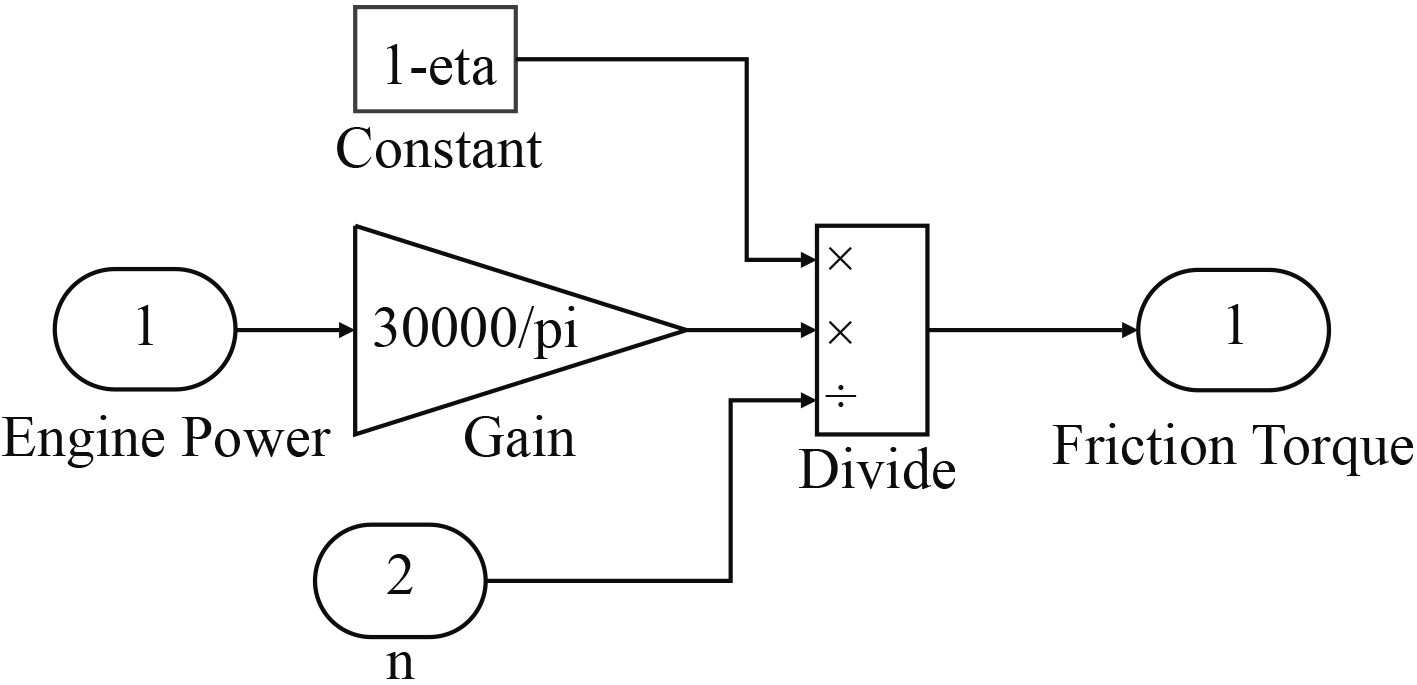图 10 齿轮箱摩擦损失仿真模型 Fig. 10 The model of friction loss of gearbox
3.5 仿真模型集成图 11 船机桨匹配仿真模型 Fig. 11 The model of ship-diesel-propeller matching
4 仿真实验与结果分析 4.1 运动方程数值解法

 \left\{ \begin{aligned} & \dot{u}={{f}_{1}}\left( t,u,v,r,\varphi ,x,y \right) {\text{，}}\\ & \dot{v}={{f}_{2}}\left( t,u,v,r,\varphi ,x,y \right) {\text{，}}\\ & \dot{r}={{f}_{3}}\left( t,u,v,r,\varphi ,x,y \right) {\text{，}}\\ & \dot{\varphi }={{f}_{4}}\left( t,u,v,r,\varphi ,x,y \right){\text{，}} \\ & \dot{x}={{f}_{5}}\left( t,u,v,r,\varphi ,x,y \right) {\text{，}}\\ & \dot{y}={{f}_{6}}\left( t,u,v,r,\varphi ,x,y \right) {\text{。}}\\ \end{aligned} \right.

tj时刻，初值为ujvjrjφjxjyj，则t=tj+1=tj+h时，各参数值的标准4阶龙格库塔公式为：

 \left\{ \begin{aligned} & {{u}_{j+1}}={{u}_{j}}+h/6\left( {{K}_{11}}+2{{K}_{12}}+2{{K}_{13}}+{{K}_{14}} \right) {\text{，}}\\ & {{v}_{j+1}}={{v}_{j}}+h/6\left( {{K}_{21}}+2{{K}_{22}}+2{{K}_{23}}+{{K}_{24}} \right) {\text{，}}\\ & {{r}_{j+1}}={{r}_{j}}+h/6\left( {{K}_{31}}+2{{K}_{32}}+2{{K}_{33}}+{{K}_{34}} \right) {\text{，}}\\ & {{\varphi }_{j+1}}={{\varphi }_{j}}+h/6\left( {{K}_{41}}+2{{K}_{42}}+2{{K}_{43}}+{{K}_{44}} \right) {\text{，}}\\ & {{x}_{j+1}}={{x}_{j}}+h/6\left( {{K}_{51}}+2{{K}_{52}}+2{{K}_{53}}+{{K}_{54}} \right){\text{，}} \\ & {{y}_{j+1}}={{y}_{j}}+h/6\left( {{K}_{61}}+2{{K}_{62}}+2{{K}_{63}}+{{K}_{64}} \right){\text{。}} \\ \end{aligned} \right.

4.2 仿真与分析

4.2.1 直航运动仿真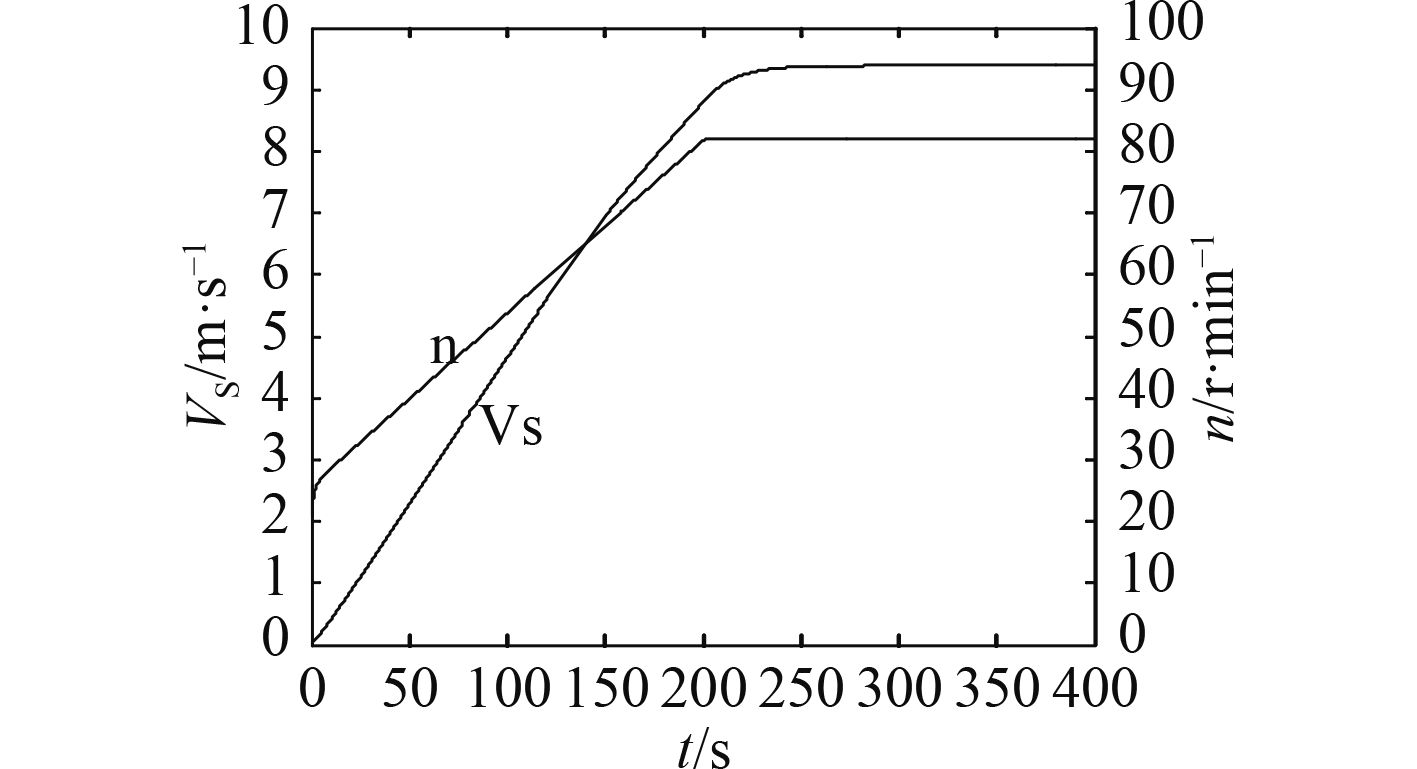图 12 船舶航速、推进器转速与时间曲线 Fig. 12 Speed of ship and rotating speed of propeller curves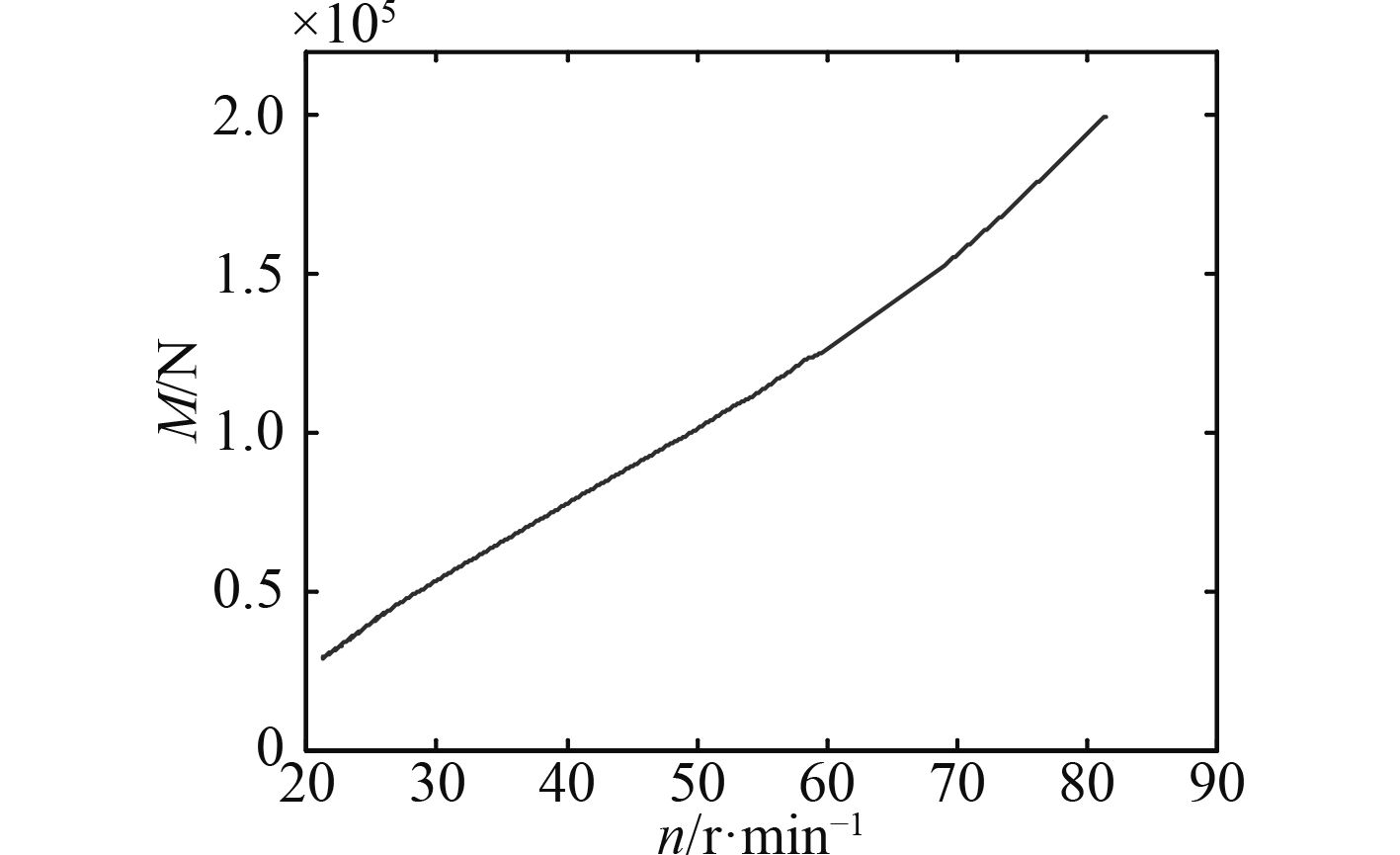图 13 直航运动时直翼桨扭矩与转速曲线 Fig. 13 Torque of propeller with rotating speed curve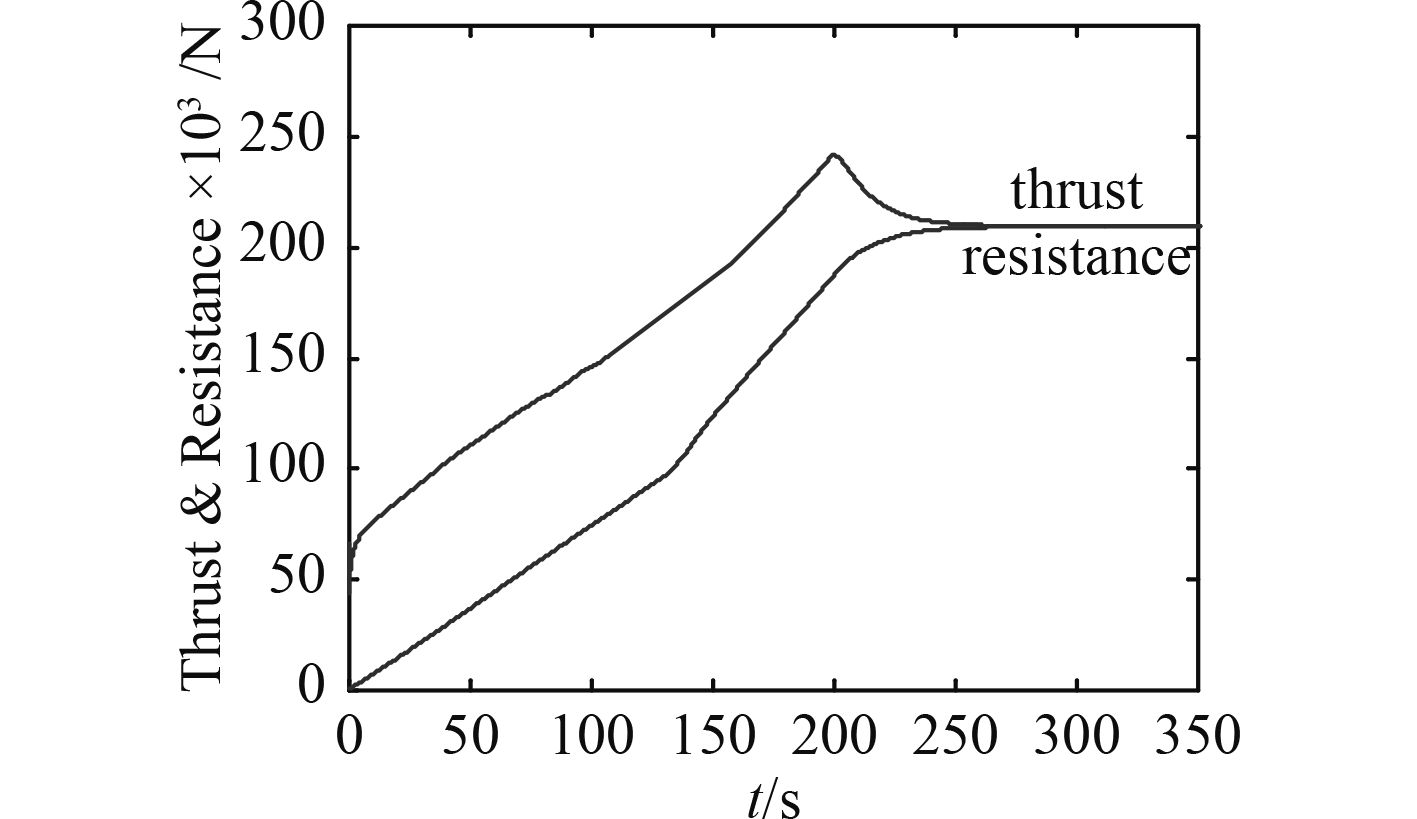图 14 直航运动时船舶推力、阻力与时间曲线 Fig. 14 Thrust of propeller and resistance of ship curves

4.2.2 回转运动仿真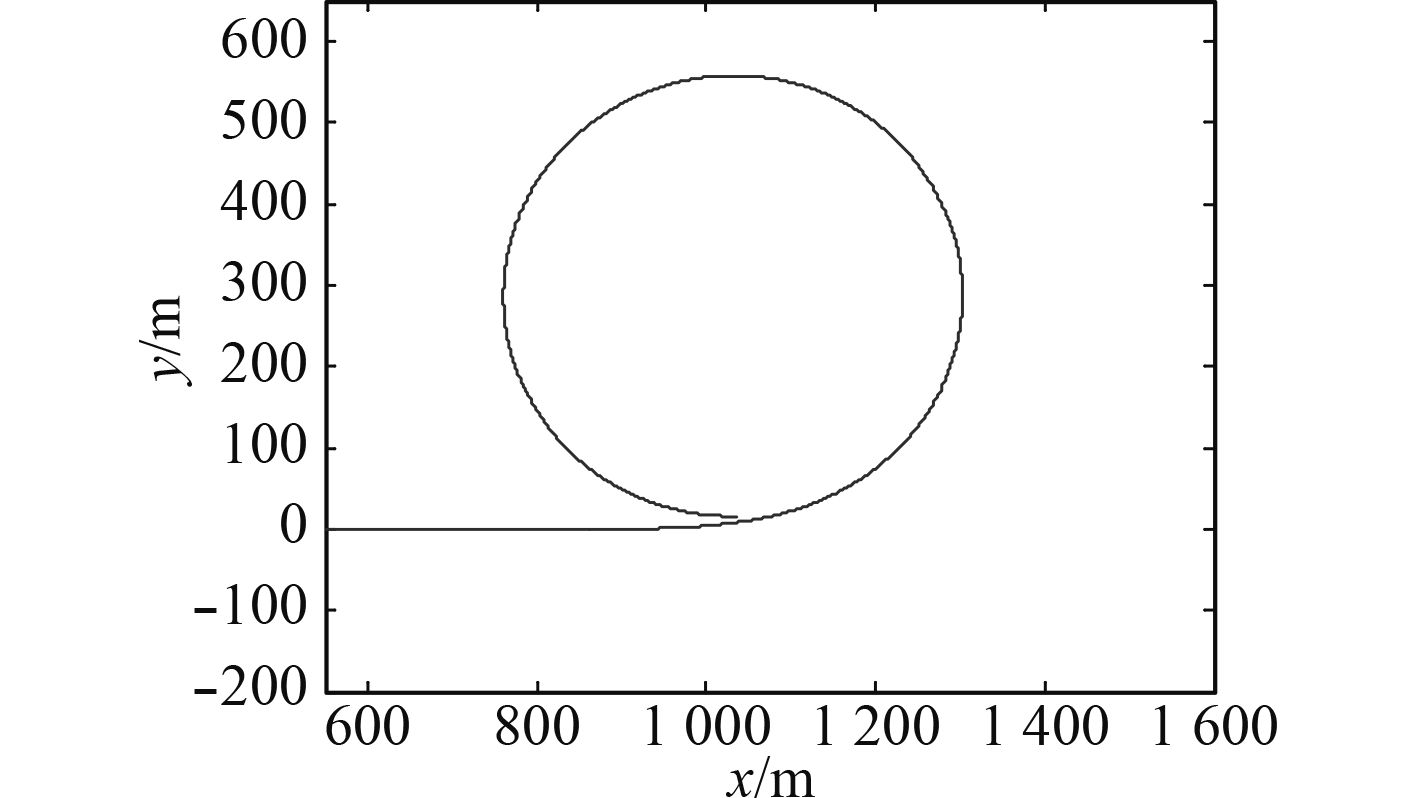图 15 船舶回转运动轨迹曲线 Fig. 15 The turning trajectory of ship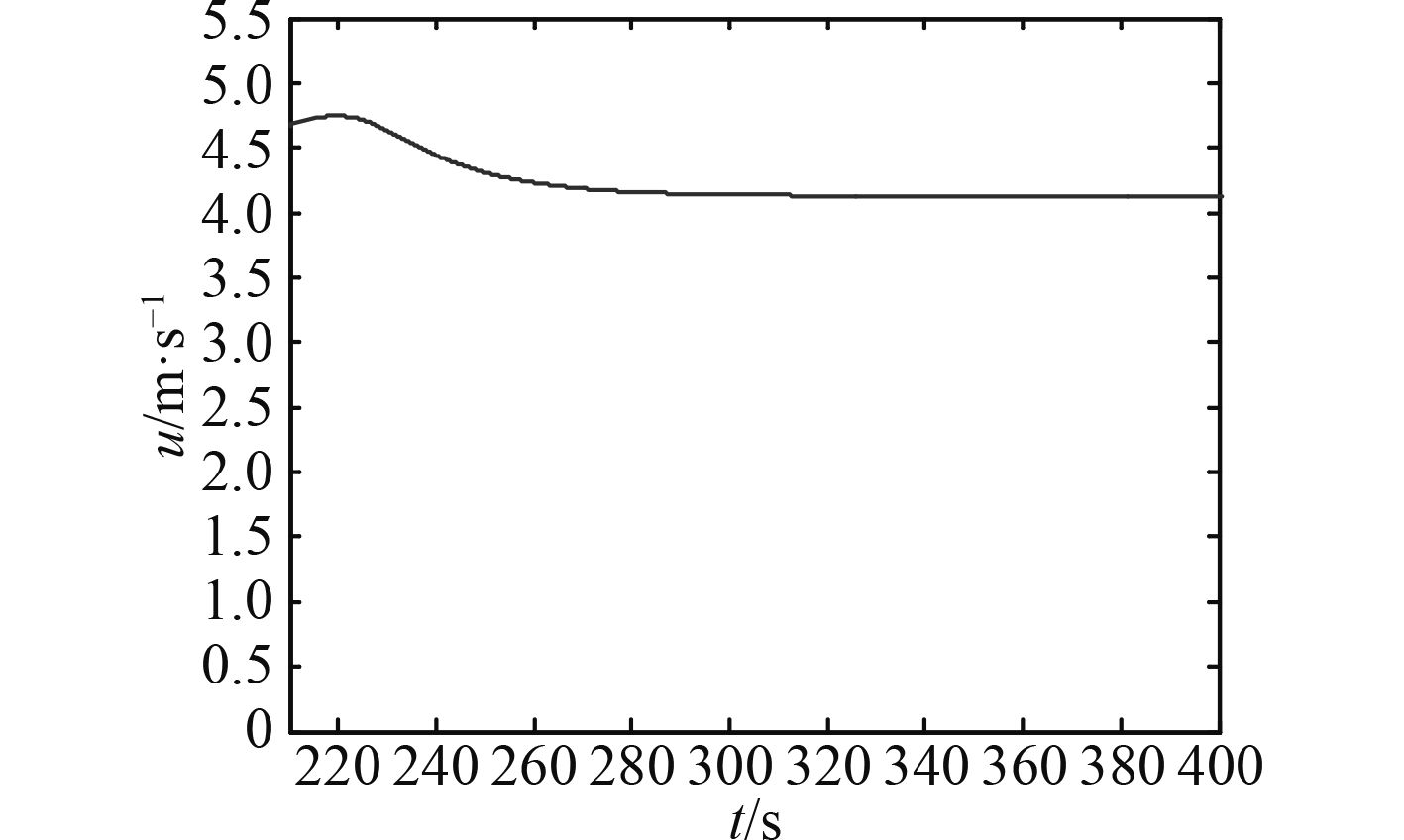图 16 船舶纵向速度曲线 Fig. 16 The curve of advance speed图 17 船舶横向速度曲线 Fig. 17 The curve of transverse speed

5 结　语

  陈先进. 摆线推进器结构及性能优化研究[D]. 杭州: 浙江大学, 2013.  覃峰, 肖金生, 詹志刚, 等. 基于遗传算法的船舶推进系统船、机、桨匹配优化设计[J]. 武汉理工大学学报, 2003, 27(1): 22-25.  王永生, 黄斌. 船舶加速过程最优机-桨联控方法仿真研究[J]. 四川大学学报, 2011, 43(1): 246-251.  邢国英. 摆线推进器任意方向角的水动力计算方法研究[J]. 水动力学研究与进展, 2005(4): 472-478. DOI:10.3969/j.issn.1000-4874.2005.04.010  高孝日. 平旋推进拖船的运动建模与仿真[D]. 大连: 大连海事大学, 2008.